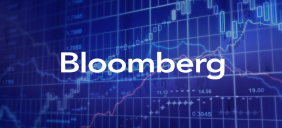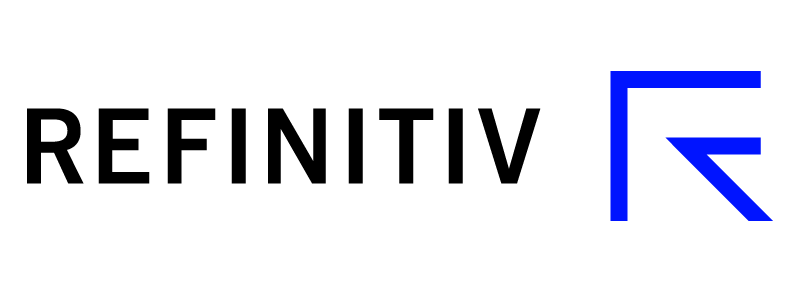# Data Vendors### Bloomberg Finance LP

EOD data is available on the following BBG Terminal tickers: KAP KX Equity, POLY KX Equity, HSBK KX Equity, HSBKY KX Equity, RUSB KX Equity, RUSE KX Equity, FAR KX Equity, IXA KX Equity, IXB KX Equity, IXE KX Equity, IXO KX Equity, IXU KX Equity, KCEL KX Equity, KCELY KX Equity, CCBN KX Equity, KSPIY KX Equity, IXD KX Equity, IXG KX Equity, IXT KX Equity, IXY KX Equity, BCCGF KX Equity, FIPO KX Equity

Tel: +9714 364 1000 / +9714 373 9400

Fax: +971 4364 1010

Email: [email protected] Level 10, Currency House, DIFC, Dubai, UAE

www.bloomberg.com### Refinitiv

Live market data is available on the following EIKON RICs: KAP=AIXN, KAPY=AIXN, HSBK=AIXN, HSBKY=AIXN, POLY=AIXN, FAR=AIXN, RUSB=AIXN, RUSE=AIXN, IXA=AIXN, IXB=AIXN, IXE=AIXN, IXO=AIXN, IXU=AIXN, XS2050933626=AIXN (MFKZ.0934), XS2050933899=AIXN (MFKZ.0926), XS1907130246=AIXN (MFKZ.1123), XS1901718335=AIXN (MFKZ.1128), CH0448036266=AIXN (KTZ.1223), KZX000000203=AIXN (ALRT.0624), KZX000000286=AIXN (ALTN.1222), KZX000000294=AIXN (FRHC.1222), XS1959301471=AIXN (QIIB.0324), KCEL=AIXN, KCELY=AIXN, XS2129651548=AIXN (BR.CCBC.0322), CCBN=AIXN, KZX000000385=AIXN (BAF.0822), KZX00000492=AIXN (DAMU.0823), RU000A101RP4=AIXN (MFKZ1.0923), RU000A101RV2=AIXN (MFKZ7.0927), RU000A101RZ3=AIXN (MFKZ1.0930), KZX000000518=AIXN (MFOK.0922), IXG=AIXN, IXY=AIXN, IXD=AIXN, IXT=AIXN, KSPI=AIXN, BCCGF=AIXN, FIPO=AIXN, KZX000000310=AIXN (TFG.1221), KZX000000609=AIXN (CHFH.1223), KZX000000567=AIXN (HCKZ1.0123)

Account Manager: Arman Kassymov

Office: +7 (727) 355 07 88 ext. 555

Mobile: +7 701 930 35 35 / +7 771 708 97 96

Email: [email protected]

050000, Almaty, Al-Farabi ave., 77/7, Esentai Tower, level 8

www.refinitiv.com

Please contact AIX Market Operations by email: [email protected] for Data Vendor inquiries